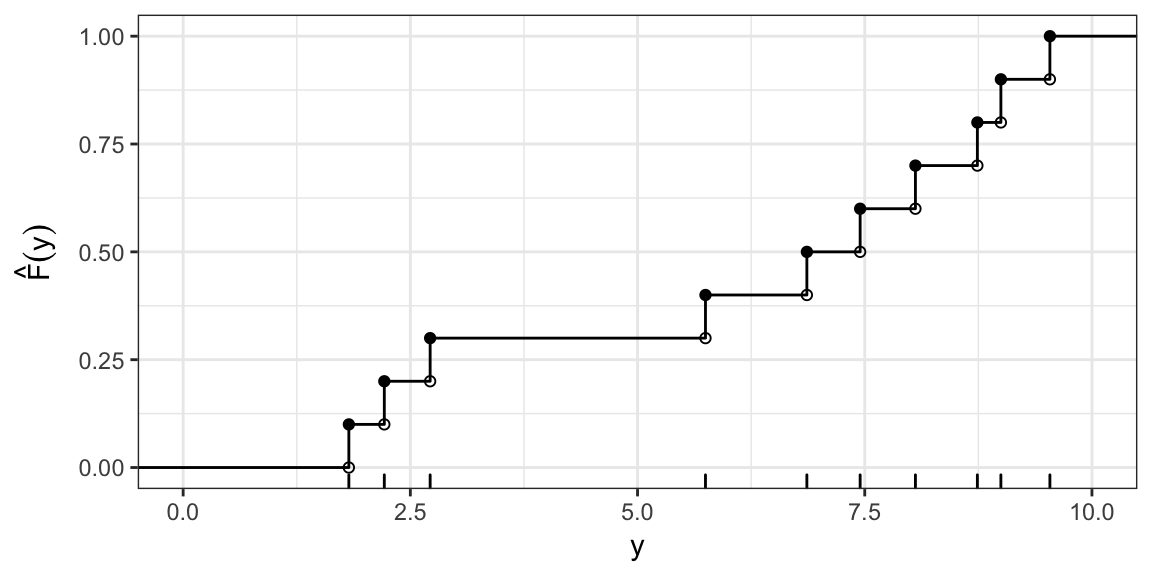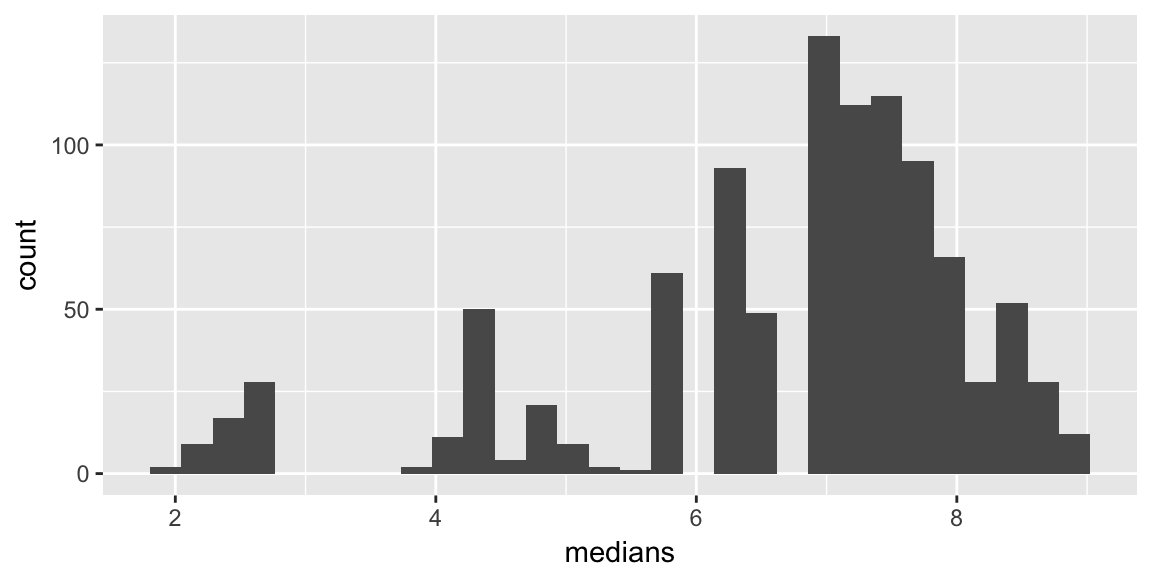# Delta Method and the Bootstrap ST551 Lecture 27

## Announcements

Lectures this week:

• Today lecture: Delta method and Bootstrap
• Weds lecture: Randomization & Permutation
• Friday lecture: Cancelled - Office hours instead

Formula Sheet The final is closed book, no note sheet. I am willing to provide some of the harder (less common) formulae.

Lab: No set material, I’ll encourage Chuan to lead a formula strategy session.

# Delta Method

## Delta Method

If the sampling distribution of a statistic converges to a Normal distribution, the Delta method, provides a way to approximate the sampling distribution of a function of a statistic.

Univariate Delta Method

If $\sqrt{n}\left(\hat{\theta} - \theta \right) \rightarrow_D N(0, \sigma^2)$

then $\sqrt{n}\left(g(\hat{\theta}) - g(\theta) \right) \rightarrow_D N(0, \sigma^2[g'( \theta]^2 )$

(As long as $$g'(\theta)$$ exists and is non-zero valued.)

## Another way of saying it

If we know, $\hat{\theta} \, \dot \sim \, N(\theta, \sigma^2)$

then,

$g(\hat{\theta}) \, \dot \sim \, N(g(\theta), \sigma^2[g'(\theta)]^2)$

The approximation can be pretty rough. I.e. just because the sample is large enough that the original statistic is reasonably Normal, doesn’t meant the transformed statistic will be.

## Example: Log Odds

Let $$Y_1, \ldots, Y_n \sim$$ Bernoulli$$(p)$$, and $$X = \sum_{i=1}^n Y_i$$.

We know $$\hat{p} = \frac{X}{n} \sim N(p, \frac{p(1 - p)}{n})$$.

We might estimate the log odds with: $\log\left(\frac{\hat{p}}{1-\hat{p}}\right)$

What is the assymptotic distribution of the estimated log odds?

## Example: Log Odds cont.

$g(p) = \log\left(\frac{p}{1-p}\right) = \log(p) - \log{(1-p)}$

## Other comments on delta method

Derived using a Taylor expansion of $$g(\hat{\theta})$$ around $$g(\theta)$$

There is also a multivariate version (useful if you need some function of two statistics, e.g. ratio of sample means)

# Bootstrap

## Bootstrap

A method to approximate the sampling distribution of a statistic

Idea:

• Recall, one way to approximate the sampling distribution of a statistic was by simulation, but you have to assume a population distribution.
• The bootstrap uses the empirical distribution function as an estimate for the population distribution, i.e relies on $\hat{F}(y) \approx F(y)$

## Example - Sampling distribution of Median by simulation

Assume a population distribution, i.e. $$Y \sim N(\mu, \sigma^2)$$

Repeat for $$k = 1, \ldots, B$$

1. Sample $$n$$ observations from $$N(\mu, \sigma^2)$$
2. Find sample median, $$m^{(k)}$$

Then the simulated sample medians, $$m^{(k)}, k = 1, \ldots, B$$ approximate the sampling distribution of the sample median.

## Example - Sampling distribution of Median by bootstrap

Estimate the population distribution from the sample, i.e. $$\hat{F}(y)$$

Repeat for $$k = 1, \ldots, B$$

1. Sample $$n$$ observations from a population with c.d.f $$\hat{F}(y)$$
2. Find sample median, $$m^{(k)}$$

Then the bootstrapped sample medians, $$m^{(k)}, k = 1, \ldots, B$$ approximate the sampling distribution of the sample median.

## Sampling from a c.d.f

You can sample from any c.d.f by sampling from a Uniform(0, 1), then transforming with the inverse c.d.f.

I.e. sample $$u_1, \ldots, u_n$$ i.i.d from Uniform(0,1), then

$y_i = F^{-1}(u_i) \quad i = 1, \ldots, n$ are distributed with c.d.f $$F(y)$$.

## In the empirical caseSampling from the ECDF is equivalent to sampling with replacement from the original sample.

## Example - Sampling distribution of Median by bootstrap

Repeat for $$k = 1, \ldots, B$$

1. Sample $$n$$ observations with replacement from $$Y_1, \ldots, Y_n$$
2. Find sample median, $$m^{(k)}$$

Then the bootstrapped sample medians, $$m^{(k)}, k = 1, \ldots, B$$ approximate the sampling distribution of the sample median.

A little more subtly: $\hat{m} - m \, \dot \sim \, \tilde{m} - \hat{m}$

## Example

Sample values: 1.8, 2.2, 2.7, 5.7, 6.9, 7.4, 8.1, 8.7, 9 and 9.5

Sample median: 7.1562828

A bootstrap resample: 1.8, 2.7, 2.7, 5.7, 6.9, 7.4, 8.1, 8.1, 8.7 and 9.5

Sample median: 7.1562828

## Many resamples## Bootstrap confidence intervals

Many methods..

A common one:

• Quantile: $$100(\alpha/2)$$ largest resampled statistic value, and $$100(1 - \alpha/2)$$ largest resampled statistic value

Relies on $$\hat{F}(y)$$ being a good estimate of the $$F(y)$$, doesn’t necessarily solve small sample problems.